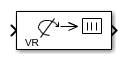Main Content

# VR Rotation to Rotation Matrix

Convert axis/angle rotation to rotation matrix

• Library:
• Simulink 3D Animation / Utilities

•## Description

The VR Rotation to Rotation Matrix block converts the axis / angle rotation representation used for defining rotations in virtual reality to a 3-by-3 rotation matrix

## Ports

### Input

expand all

Input rotation, specified as a 4-element vector in axis/angle notation,. The first three elements specify the axis of rotation and the fourth element specifies the angle.

Data Types: `single` | `double`

### Output

expand all

3D rotation, returned as a 3-by-3 columnwise defined matrix, also known as a direction cosine matrix.

A representation of a three-dimensional spherical rotation as a 3-by-3 real, orthogonal matrix R: RTR = RRT = I, where I is the 3-by-3 identity and RT is the transpose of R. This matrix is also known as the direction cosine matrix (DCM). The DCM is the orientation of the object in space, relative to its parent node.

`$R=\left(\begin{array}{ccc}{R}_{11}& {R}_{12}& {R}_{13}\\ {R}_{21}& {R}_{22}& {R}_{23}\\ {R}_{31}& {R}_{32}& {R}_{33}\end{array}\right)=\left(\begin{array}{ccc}{R}_{xx}& {R}_{xy}& {R}_{xz}\\ {R}_{yx}& {R}_{yy}& {R}_{yz}\\ {R}_{zx}& {R}_{zy}& {R}_{zz}\end{array}\right)$`

Data Types: `single` | `double`

## Parameters

expand all

Input signal value is considered to be zero if it is equal to or lower than the value set in this parameter. By default, the parameter is set to ε = 1e-12.

## See Also

Introduced in R2019a

## Support평가판 신청